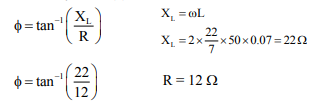Deepak Scored 45->99%ile with Bounce Back Crack Course. You can do it too!

# A 0.07 H inductor

Question:

A 0.07 H inductor and a 12 resistor are connected in series to a 220 V, 50 Hz ac source. The approximate current in the circuit and the phase angle between current and source voltage are respectively. [Take $\pi$ as $\frac{22}{7}$ ]

1. $8.8 \mathrm{~A}$ and $\tan ^{-1}\left(\frac{11}{6}\right)$

2. $88 \mathrm{~A}$ and $\tan ^{-1}\left(\frac{11}{6}\right)$

3. $0.88 \mathrm{~A}$ and $\tan ^{-1}\left(\frac{11}{6}\right)$

4. $8.8 \mathrm{~A}$ and $\tan ^{-1}\left(\frac{6}{11}\right)$

Correct Option: 1,

Solution:$\phi=\tan ^{-1}\left(\frac{11}{6}\right)$

$Z=\sqrt{\mathrm{X}_{\mathrm{L}}^{2}+\mathrm{R}^{2}}=25.059$

$\mathrm{I}=\frac{\mathrm{V}}{\mathrm{Z}}=\frac{220}{25.059}=8.77 \mathrm{~A}$